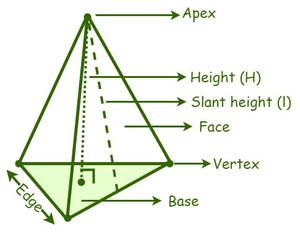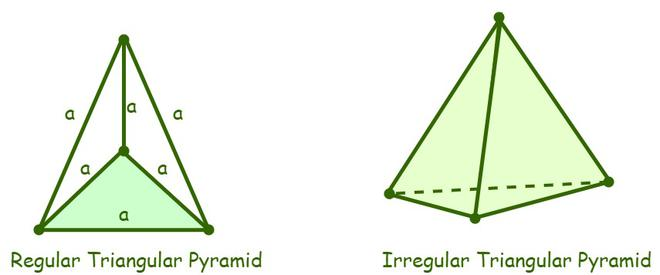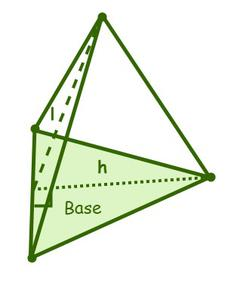Open in App
Not now

# Triangular Pyramid Formula

• Last Updated : 29 Jul, 2022

In geometry, a pyramid is a polyhedron, which is a three-dimensional solid figure whose base is a polygon and all its triangular faces join at a common point called the apex or vertex. The pyramids of Egypt; rooftops; camping tents; telephone towers, etc. are some real-life examples of pyramids. A pyramid is classified into various kinds on the basis of the shape of the base, such as a triangular pyramid, a square pyramid, a pentagonal pyramid, a hexagonal pyramid, etc. An apex is a meeting point of the lateral surfaces or the side faces of a pyramid. The perpendicular distance from the apex of a pyramid to the center of its base is the height or altitude of a pyramid. The perpendicular distance between the apex and the base of a lateral surface slant height of a pyramid.## Triangular Pyramid

A triangular pyramid is a pyramid that has a triangle as its base. It is also known as a tetrahedron and has three triangular-shaped faces and one triangular base, where the triangular base can be scalar, isosceles, or an equilateral triangle. A triangular is further classified into three types i.e., a regular triangular pyramid, an irregular triangular pyramid, and a right triangular pyramid.

• Regular triangular pyramid: A triangular pyramid whose four faces are equilateral triangles is called a regular triangular pyramid. As the pyramid is made up of equilateral triangles, the measure of all its internal angles is 60°.• Irregular Triangular pyramid: An irregular triangular pyramid is one whose edges of the base are not equal, i.e., the base of an irregular triangular pyramid is either a scalene triangle or an isosceles triangle. All triangular pyramids are assumed to be regular triangular pyramids unless a triangular pyramid is specifically mentioned as irregular.
• Right Triangular pyramid: A right triangular pyramid is one whose base is a right-angled triangle and whose apex is aligned above the center of the base.

### Triangular Pyramid Formula

There are two formulas for a triangular pyramid: the surface area of a triangular pyramid and the volume of a triangular pyramid.

Surface area of a Triangular Pyramid

The surface area of a pyramid has two types of surface areas, namely: the lateral surface area and the total surface area, where the surface area of a pyramid is the sum of the areas of the lateral surfaces, or side faces, and the base area of a pyramid.Lateral surface area of a triangular pyramid (LSA) = ½ × perimeter × slant height

The total surface area of a pyramid (TSA) = Lateral surface area of the pyramid + Area of the base

So, TSA = ½ × perimeter × slant height + ½ × base × height

Total surface area (TSA) of a triangular pyramid = ½ ×  P ×  l + ½ bh

where,

P is the perimeter of the base,

l is the slant height of the pyramid,

b is the base of the base triangle, and

h is the height of the pyramid

Volume of a Triangular Pyramid

The volume of a pyramid is the total space enclosed between all the faces of a pyramid. The volume of a pyramid is generally represented by the letter “V”, and its formula is equal to one-third of the product of the base area and the height of the pyramid.

The formula for the volume of a pyramid is given as follows,

Volume of a Triangular Pyramid = 1/3 × base area × height

V = 1/3 × AH cubic units

where,

V is the volume of the pyramid,

A is the area of the base of a pyramid, and

H is the height or altitude of a pyramid.

The formula for the volume of a regular triangular pyramid is given as follows

Volume of a regular triangular pyramid = a3/6√2 cubic units

Where a is the length of the edges.

### Practise Problem  based on Triangular Pyramid Formula

Problem 1: Determine the volume of a triangular pyramid whose base area and height are 50 cm2 and 12 cm, respectively.

Solution:

Given data,

Area of the triangular base = 100 cm2

The height of the pyramid = 12 cm

We know that,

The volume of a triangular pyramid (V) = 1/3 × Area of triangular base × Height

V = 1/3 × 50 × 12 = 200 cm3

Hence, the volume of the given triangular pyramid is 200 cm3.

Problem 2: Find the total surface area of a regular triangular pyramid when the length of each edge is 8 inches.

Solution:

Given data,

The length of each edge of a regular triangular pyramid (a) = 8 inches

We know that,

The total surface area of a regular triangular pyramid = √3a2

⇒ TSA = √3 × 82

= 64√3 = 110.851 sq. in

Hence, the total surface area of a regular triangular pyramid is 110.851 sq. in.

Problem 3: Determine the volume of a regular triangular pyramid when the length of the edge is 10 cm.

Solution:

Given data,

The length of each edge of a regular triangular pyramid (a) = 10 cm

We know that,

The volume of a regular triangular pyramid = a3/6√2

⇒ V = (10)3/6√2

= 1000/6√2 = 117.85 cm3

Hence, the volume of a regular triangular pyramid is 117.85 cu. cm.

Problem 4: Find the slant height of the triangular pyramid if its lateral surface area is 600 sq. in. and the perimeter of the base is 60 inches.

Solution:

Given data,

The lateral surface area = 600 sq. in

The perimeter of the base = 60 inches

We know that,

The lateral surface area = ½ × perimeter × slant height

600 = ½ × 60 × l

l = 600/30 = 20 inches

Hence, the slant height of the given pyramid is 20 inches.

Problem 5: Determine the total surface area of a triangular pyramid whose base area is 28 sq. cm, the perimeter of the triangle is 18 cm, and the slant height of the pyramid is 20 cm.

Solution:

Given data,

Area of the triangular base = 28 cm2

The slant height (l) = 20 cm

Perimeter (P) = 18 cm

We know that,

The total surface area (TSA) of a triangular pyramid = ½ × perimeter × slant height + Base area

⇒ TSA = ½ × 18 × 20 + 28

= 180 + 28 = 208 sq. cm

Hence, the total surface area of the given pyramid is 208 sq. cm.

Question 1: What is the definition of a triangular pyramid?

A triangular pyramid is a geometric shape that has a triangular base and three triangular faces, having a common vertex.

Question 2: How many faces and vertices do a triangular pyramid have?

Triangular pyramid has four faces and  four vertices. One vertex is common to all three faces of the pyramid.

Question 3: What are the types of triangular pyramids?